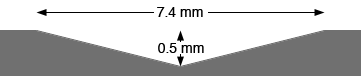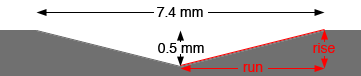SEARCH HOMEMath Central Quandaries & QueriesQuestion from William: What is the slope of a hole that is 7.4 mm in dia by .5mm in depth.William,

My understanding of your question is that you have drilled a shallow conical hole in some metal. The hole os 7.4 mm in diameter and 0.5 mm deep in the center and you want to know the slope of the side of the hole.The slope of a line is the rise divided by the run as illustrated in the diagram below.In this case the rise is 0.5 mm and the run is 7.4/2 = 3.7 mm and hence the slope is 0.5/3.7 = 0.135.

Let me know if I have not interpreted your question correctly,
PennyMath Central is supported by the University of Regina and The Pacific Institute for the Mathematical Sciences.